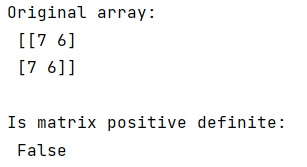# Find out if matrix is positive definite with numpy

Learn, how to find out if matrix is positive definite with numpy in Python?
Submitted by Pranit Sharma, on January 14, 2023

NumPy is an abbreviated form of Numerical Python. It is used for different types of scientific operations in python. Numpy is a vast library in python which is used for almost every kind of scientific or mathematical operation. It is itself an array which is a collection of various methods and functions for processing the arrays.

## Positive Definite Matrix

A positive definite matrix is a symmetric matrix where every eigenvalue is positive. Suppose that there is a vector z and this z will have a certain direction. If we multiply matrix M with z, z will no longer point in the same direction. The direction of z is transformed by M. If M is a positive definite matrix, then the new direction will always lie in "the same general" direction. It will not reverse (= more than a 90-degree angle change) the original direction.

## Checking whether if a matrix is positive definite

For this purpose, we will check if all the eigenvalues of the matrix are positive, if so, the matrix is positive definite.

But, for real matrices, the tests for positive eigenvalues and positive-leading terms in np.linalg.cholesky only applies if the matrix is symmetric. So first one needs to test if the matrix is symmetric and then apply one of those methods

Let us understand with the help of an example,

## Python code to check whether if a matrix is positive definite with numpy

```# Import numpy
import numpy as np

# Creating numpy array
arr = np.array([[7, 6],[7, 6]])

# Display original array
print("Original array:\n",arr,"\n")

# Check all eigen value
res = np.all(np.linalg.eigvals(arr) > 0)

# Display result
if(res):
print("Is matrix positive definite:\n",res)
else:
print("Is matrix positive definite:\n", res)
```

### Output

The output of the above program is: